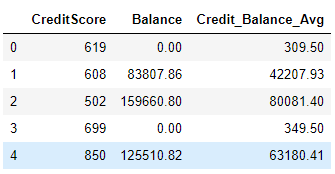The Pandas apply function is used to apply different functions on Pandas dataframes and series. The Pandas apply function is one of the few Python functions that can operate on both series and dataframes. That’s because the `apply` function is designed to operate on each row of a Pandas column or dataframe. In this tutorial, you’ll see how to use the Pandas apply function to perform different tasks on series and dataframes. Recall, a series is basically a single column of data that either stands by itself or is extracted from a parent dataframe.

## Installing Required Libraries

You’ll need both the Pandas library and the Numpy library to execute the scripts in this tutorial. We’ll be demonstrating how to use a few NumPy functions with the Pandas apply function, so that’s why we recommend you get it, too. Execute the following script in your command terminal to install the Pandas and the NumPy libraries.

```\$ pip install pandas
\$ pip install numpy
```

### The Dataset

We’ll be using the bank customer churn dataset for several examples in this tutorial. The dataset is available at this Github link.

The following script imports the dataset and displays its first 5 rows.

``````import pandas as pd
import numpy as np
data_path = "https://raw.githubusercontent.com/aniruddhgoteti/Bank-Customer-Churn-Modelling/master/data.csv.csv"

Output: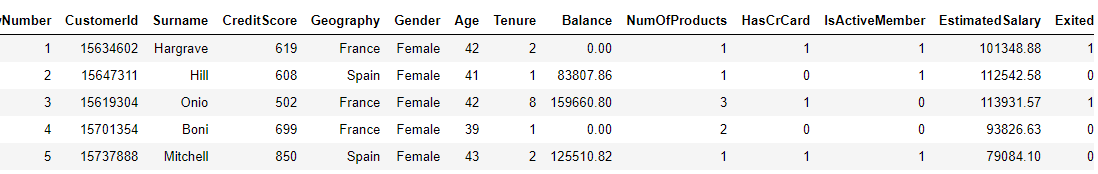The first 13 columns of the dataset contain information about different customers. The 14th column shows whether or not the customer left the bank. This is called customer churn.

### Pandas Apply Function with Series

As we said earlier, the Pandas apply function can be applied to both series and dataframes. In this section, we’ll see how to apply the Pandas apply function to a series.

In the following script, we find the length of the strings in the Surname column using the apply function. To do so, we have to first select the column name, followed by a dot operator and then the `apply` function. The function that we want to apply on the Surname column is passed as a parameter to the apply function. The `len` function from the Python library can be used to find lengths of strings, therefore we simply pass the Python `len` function to the apply function. The this example, the `apply` function applies the `len` function on each row in the series and creates a new column, Surname_Length, where we store the length of strings in the Surname column.

``````bank_data["Surname_Length"] = bank_data["Surname"].apply(len)

In the output, you see both the Surname and the Surname_Length columns as shown below.

Output:In addition to using Python’s default functions, such as `len`, you can also pass the Numpy library’s function to the apply function as shown below. The following script rounds up the values in the Balance column of the dataset using NumPy’s `np.ceil` function.

``````bank_data["Balance_Rounded"] = bank_data["Balance"].apply(np.ceil)

Output: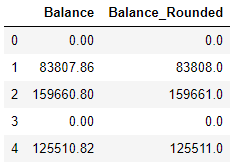You can also pass your own lambda functions to the Pandas `apply` function. For example, the following script uses a lambda function inside the apply function to convert the values in the Balance column from dollars to euros, assuming 1 Dollar = 0.90 Euros (approximately). This is great for converting units, like feet to meters or pounds to kilograms.

``````bank_data["Balance_Euros"] = bank_data["Balance"].apply(lambda x: x* 0.9)

Output: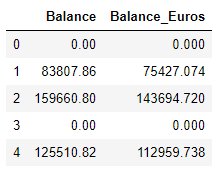Finally, you can define full custom functions and use these custom fuctions inside the `apply` function.

In the following script we define a function, `double_credit`, which doubles any value passed to it as a parameter.

``````def double_credit(credit_value):
return credit_value * 2``````

Next, we use the apply function to apply our custom `double_credit` function to each row of the CreditScore column. The result is stored in a new column Double_Credit. Finally, the first five rows of the CreditScore and Double_Credit columns are displayed in the output. You can imagine how powerful it is to be able to quickly apply your own custom Python functions to individual series or columns in a Pandas Dataframe without having to manually iterate each row.

``````bank_data["Double_Credit"] = bank_data["CreditScore"].apply(double_credit)

Output:I put together a Python Developer Kit with over 100 pre-built Python scripts covering data structures, Pandas, NumPy, Seaborn, machine learning, file processing, web scraping and a whole lot more - and I want you to have it for free. Enter your email address below and I'll send a copy your way.

Yes, I'll take a free Python Developer Kit

### Pandas Apply Function with Dataframes

Now let’s show you how the apply function can be applied to Pandas dataframes. It’s important to mention that the apply function can be applied along both x-axes (i.e. along rows) and along columns.

Let’s first filter two columns: CreditScore and Balance from our dataset. We will be using these two columns to see how the apply function can be applied on dataframes.

``bank_data[["CreditScore", "Balance"]].head()``

Output: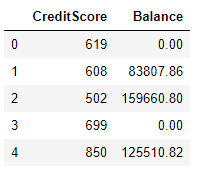Now, we’ll find the mean of all the data in each column. To do so, you can use NumPy’s `np.mean` function. To find the mean value of all rows in each column, pass `axis = 0` as a parameter to the `apply` function, like this:

``bank_data[["CreditScore", "Balance"]].apply(np.mean, axis = 0)``

Output:

``````CreditScore      650.528800
Balance        76485.889288
dtype: float64``````

Similarly, the following script finds the maximum values from both the CreditScore and Balance columns.

``bank_data[["CreditScore", "Balance"]].apply(max, axis = 0)``

Output:

``````CreditScore       850.00
Balance        250898.09
dtype: float64``````

You can also find the maximum values across columns by setting `axis = 1`. This will return the maximum value from all the columns for each row in your dataframe.

``bank_data[["CreditScore", "Balance"]].apply(max, axis = 1)``

Output:

``````0          619.00
1        83807.86
2       159660.80
3          699.00
4       125510.82
...
9995       771.00
9996     57369.61
9997       709.00
9998     75075.31
9999    130142.79
Length: 10000, dtype: float64``````

The output shows that in the first row, the value of CreditScore (619) is greater than the value in the Balance column. Hence, 619 is returned in the output.

Just like with series, you can use lambda functions inside the apply function. The following `apply` function example adds the values in the CreditScore and Balance columns.

``````bank_data["Credit_Balance"] = bank_data[["CreditScore", "Balance"]].apply(lambda x: x["CreditScore"] + x["Balance"], axis = 1)

Output:The script above is just an example of a lambda function for the sake of explanation. Practically, you would add values in two columns like this:

``````bank_data["Credit_Balance2"] =  bank_data["CreditScore"] + bank_data["Balance"]

Output: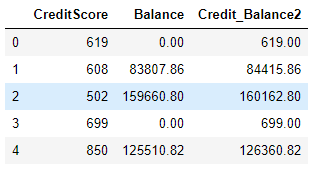Finally, user defined functions can be passed to the apply function to perform complex operations on an entire Pandas dataframe. For example the `get_mean` function in the following script returns the average of the values in the CreditScore and Balance columns of the dataframe it’s given as a parameter.

``````def get_mean(df):
return ((df["CreditScore"] + df["Balance"]) / 2)``````

Once we’ve defined our custom function, the following script applies our `get_mean()` function to the bank_data dataframe via the `apply` function and returns the average of the values in the CreditScore and Balance columns.

``````bank_data["Credit_Balance_Avg"] = bank_data[["CreditScore", "Balance"]].apply(get_mean, axis = 1)